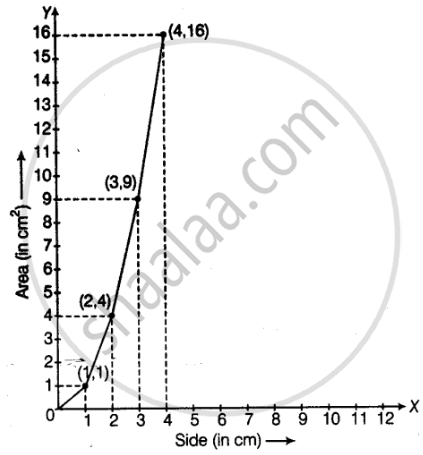# Make a line graph for the area of a square as per the given table. Side (in cm) 1 2 3 4 Area (in cm2) 1 4 9 16 Is it a linear graph? - Mathematics

Graph

Make a line graph for the area of a square as per the given table.

 Side (in cm) 1 2 3 4 Area (in cm2) 1 4 9 16

Is it a linear graph?

#### SolutionYes, it is linear graph.

Concept: Linear Graphs
Is there an error in this question or solution?

#### APPEARS IN

NCERT Mathematics Exemplar Class 8
Chapter 12 Introduct To Graphs
Exercise | Q 45 | Page 380